﻿ 基于分层组织的超声时频信号对比分析
«上一篇文章快速检索 高级检索

 应用科技2018, Vol. 45Issue (5): 38-44, 50  DOI: 10.11991/yykj.2017110060

### 引用本文KANG Weixin, GONG Xue, LI Haifeng. Contrastive analysis of ultrasonic time-frequency signals in laminated tissues[J]. Applied Science and Technology, 2018, 45(5), 38-44, 50. DOI: 10.11991/yykj.201711006.### 文章历史

Contrastive analysis of ultrasonic time-frequency signals in laminated tissues
KANG Weixin, GONG Xue, LI Haifeng
College of Information and Communication Engineering, Harbin Engineering University, Harbin 150001, China
Abstract: Aiming at the ultrasound testing for biological tissue microstructure, based on the propagation mechanism of ultrasonic transmission method in biological tissue, this paper presents a time-frequency domain signal transmission model of laminated soft tissues. In order to describe the effect of biological tissue structure’s change on the ultrasonic detection signal, and to compare the sensitivity of ultrasonic time-frequency domain signal to changes in the microstructure of tissue, the time-frequency domain signal was used to detect the laminated structure, and the maximum amplitude method and the energy-scaled amplitude were adopted to process the received signals. The simulation experiments show that the time domain signal is more sensitive to the change of tissue composition, and better for the detection performance of biological microstructure, while the energy-scaled method has higher resolution of signal changes.
Keywords: biological tissue    microstructure    ultrasonic testing    hierarchical model    time-frequency domain signal    maximum amplitude method    energy-scaled amplitude    recognition rate

1 超声传播模型 1.1 传播衰减

 $\alpha (f) = {\alpha _0}{(f/{f_0})^n}$

 $h(r,t) = \sum\limits_{i = 1}^N {{A_i}(t)} \delta (t - {\tau _i})$

 $x(t) = [1 - \cos (\frac{{2{\rm{\pi }} \cdot {f_0} \cdot t}}{m})] \cdot \sin (2{\rm{\pi }} \cdot {f_0} \cdot t)$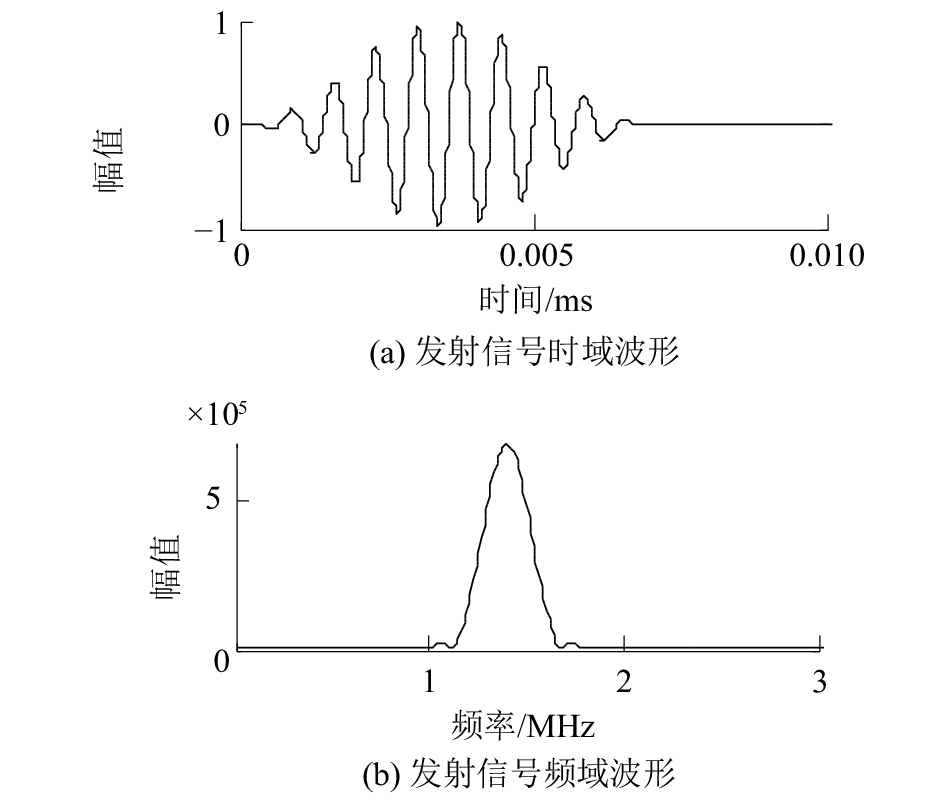Download: 图 1 发射信号

 $s(r,t) = x(t)h(r,t)$ (1)
 $S(w) = X(w)H(w) = X(w)\exp ( - \alpha (f)d)$ (2)
1.2 分层组织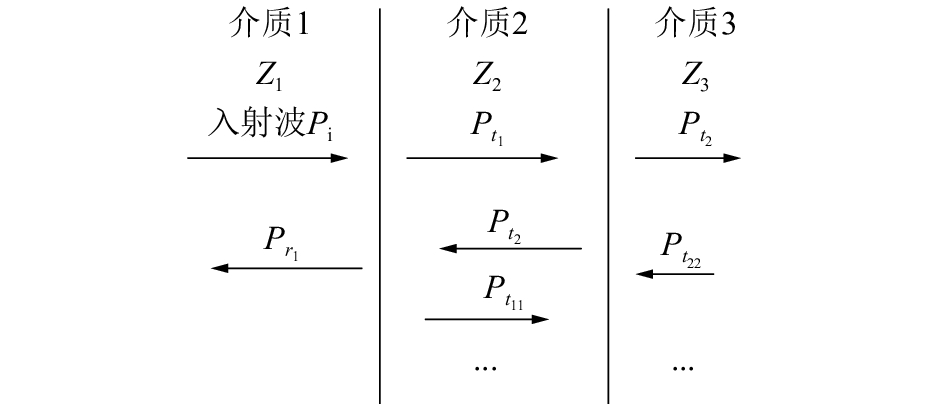Download: 图 2 超声遇界面透射、反射示意

 ${t_{{p_{_{1}}}}} = \frac{{{p_{{t_1}}}}}{{{p_i}}} = \frac{{2{z_2}}}{{{z_1} + {z_2}}}$
 ${t_{{p_{_{2}}}}} = \frac{{{p_{{t_2}}}}}{{{p_{{t_1}}}}} = \frac{{2{z_3}}}{{{z_2} + {z_3}}}$
2 信号测量 2.1 最大值法

 $I(t) = \max \{ \left| {s(t)} \right|\}$
 $J(\omega ) = \max \{ \left| {S(\omega )} \right|\}$

 $A = \left[ {{I_1}(t),{I_2}(t),{I_3}(t),{I_4}(t), \cdots \cdots } \right]$
 $B = \left[ {{J_1}(\omega ),{J_2}(\omega ),{J_3}(\omega ),{J_4}(\omega ), \cdots \cdots } \right]$

 ${n_{N - 1}} = \frac{{\max \{ \left| {{s_N}(t)} \right|\} - \max \{ \left| {{s_{N - 1}}(t)} \right|}}{{\max \{ \left| {{s_N}(t)} \right|\} }}$

 ${n_{N - 1}} = \frac{{\max \{ \left| {{s_{N - 1}}(t)} \right|\} - \max \{ \left| {{s_N}(t)} \right|}}{{\max \{ \left| {{s_{N - 1}}(t)} \right|\} }}$

 $N = \left[ {{n_1},{n_2},{n_3},{n_4}, \cdots \cdots } \right]$
 $M = \left[ {{m_1},{m_2},{m_3},{m_4}, \cdots \cdots } \right]$
2.2 能量比较法

 $E(t) = {\int_{{t_1}}^{{t_2}} {s(t)} ^2}{\rm{d}}t$

 $E(w) = {\int_{{w_1}}^{{w_2}} {S(w)} ^2}{\rm{d}}w$

 $C = \left[ {{E_1}(t),{E_2}(t),{E_3}(t),{E_4}(t), \cdots \cdots } \right]$
 $D = \left[ {{E_1}(w),{E_2}(w),{E_3}(w),{E_4}(w), \cdots \cdots } \right]$

 $P = \left[ {{p_1},{p_2},{p_3},{p_4}, \cdots \cdots } \right]$
 $Q = \left[ {{q_1},{q_2},{q_3},{q_4}, \cdots \cdots } \right]$
3 仿真实验

3.1 单层组织表 1 单层组织的特性参数值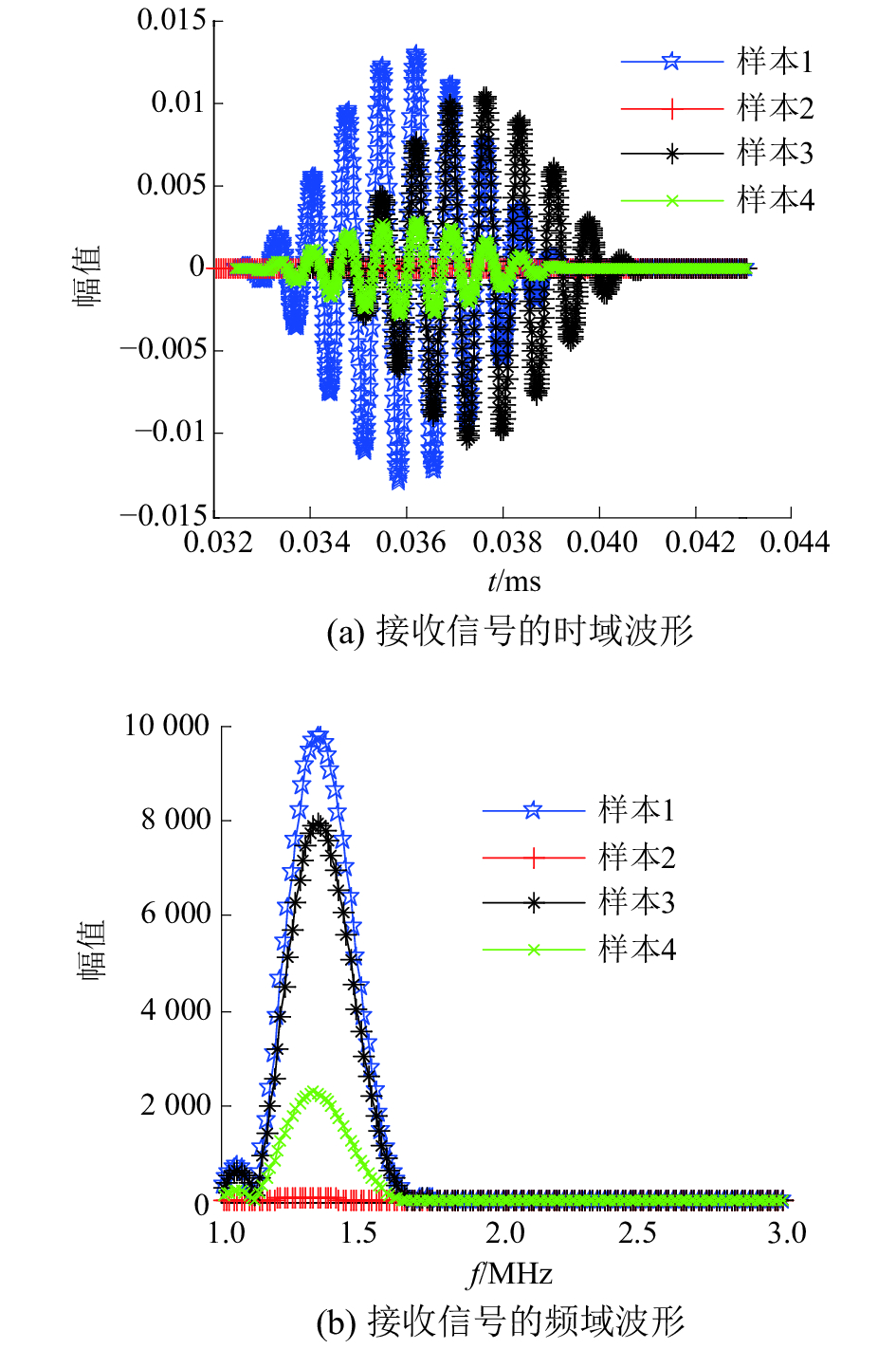Download: 图 3 单层组织的接收信号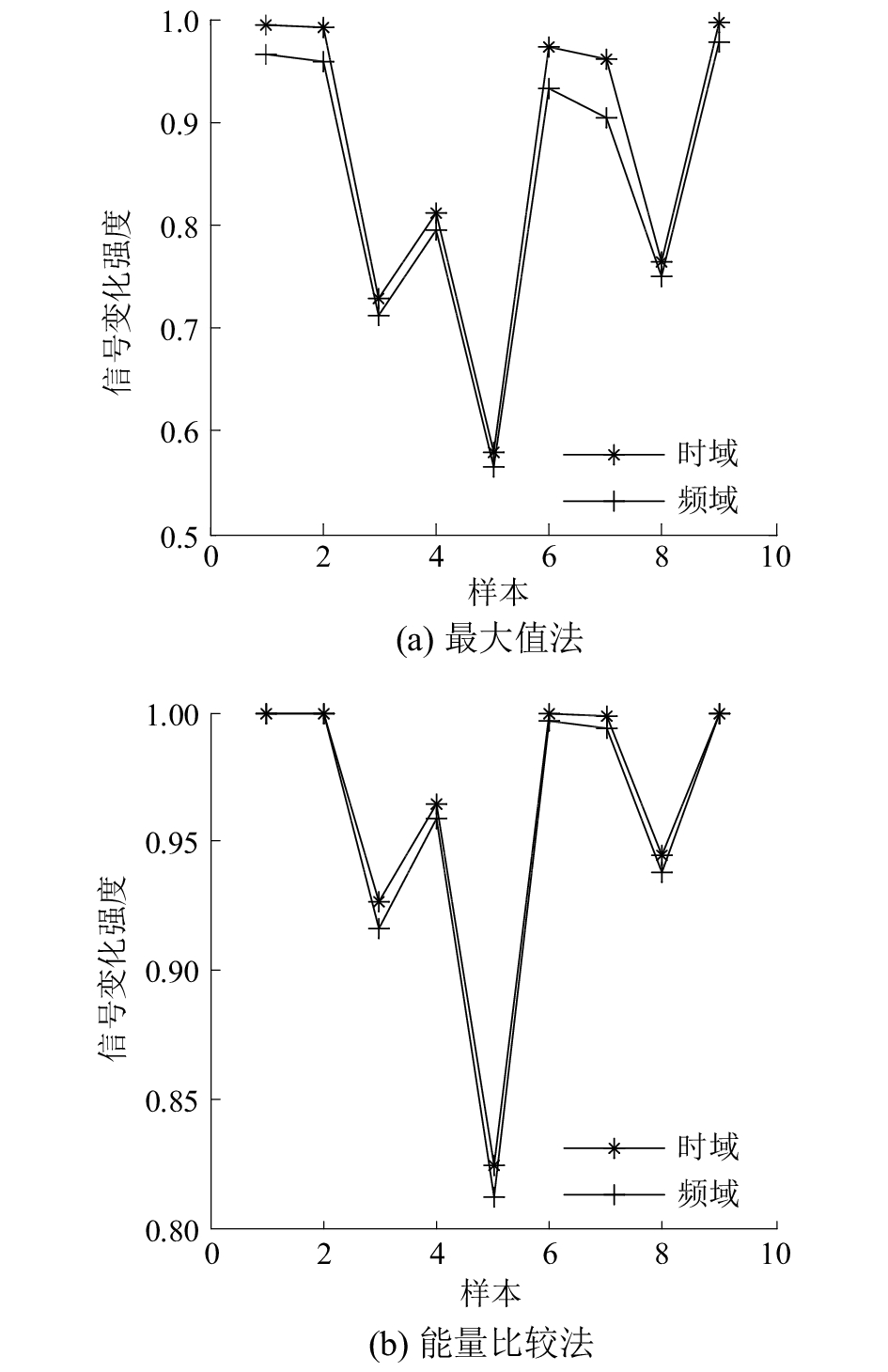Download: 图 4 信号变化示意（单层）

3.2 双层组织表 2 双层组织的特性参数值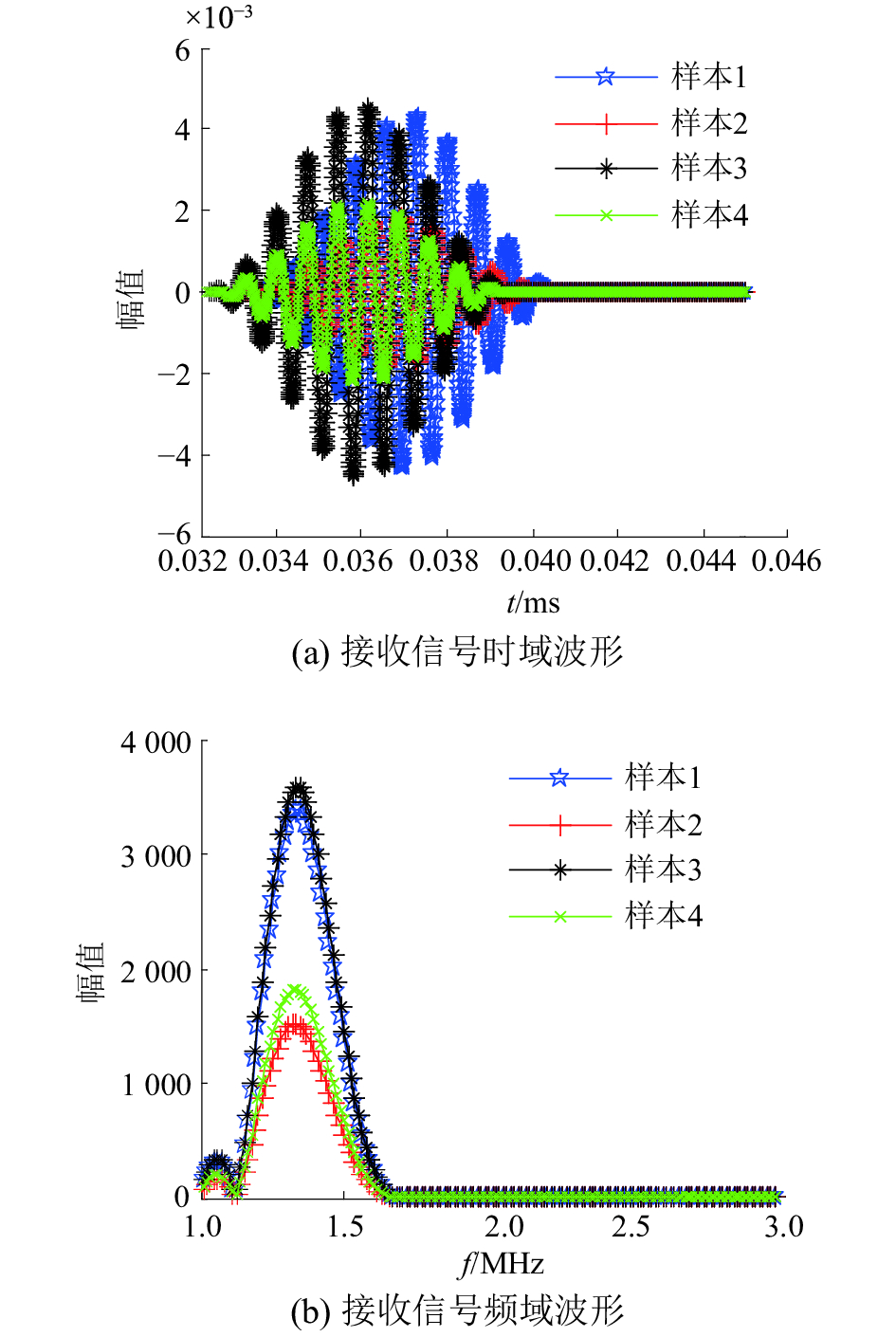Download: 图 5 双层组织的接收信号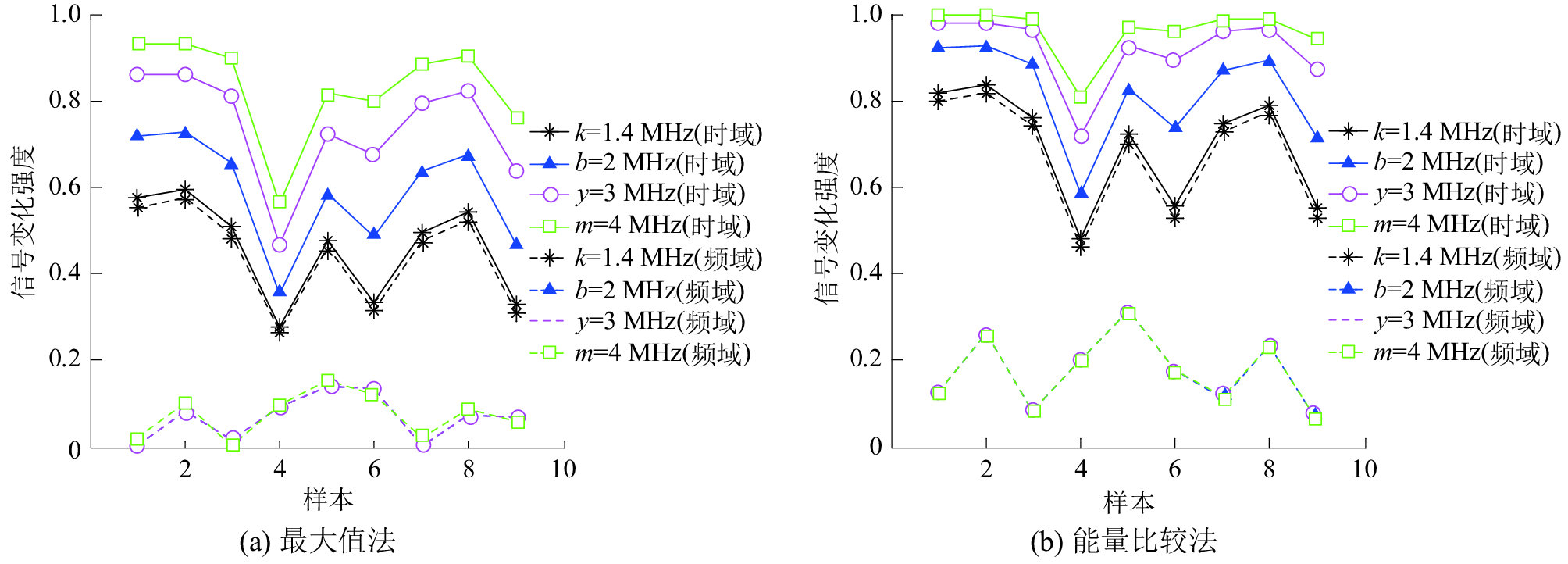Download: 图 6 信号变化示意（双层）

3.3 三层组织表 3 三层组织的特性参数值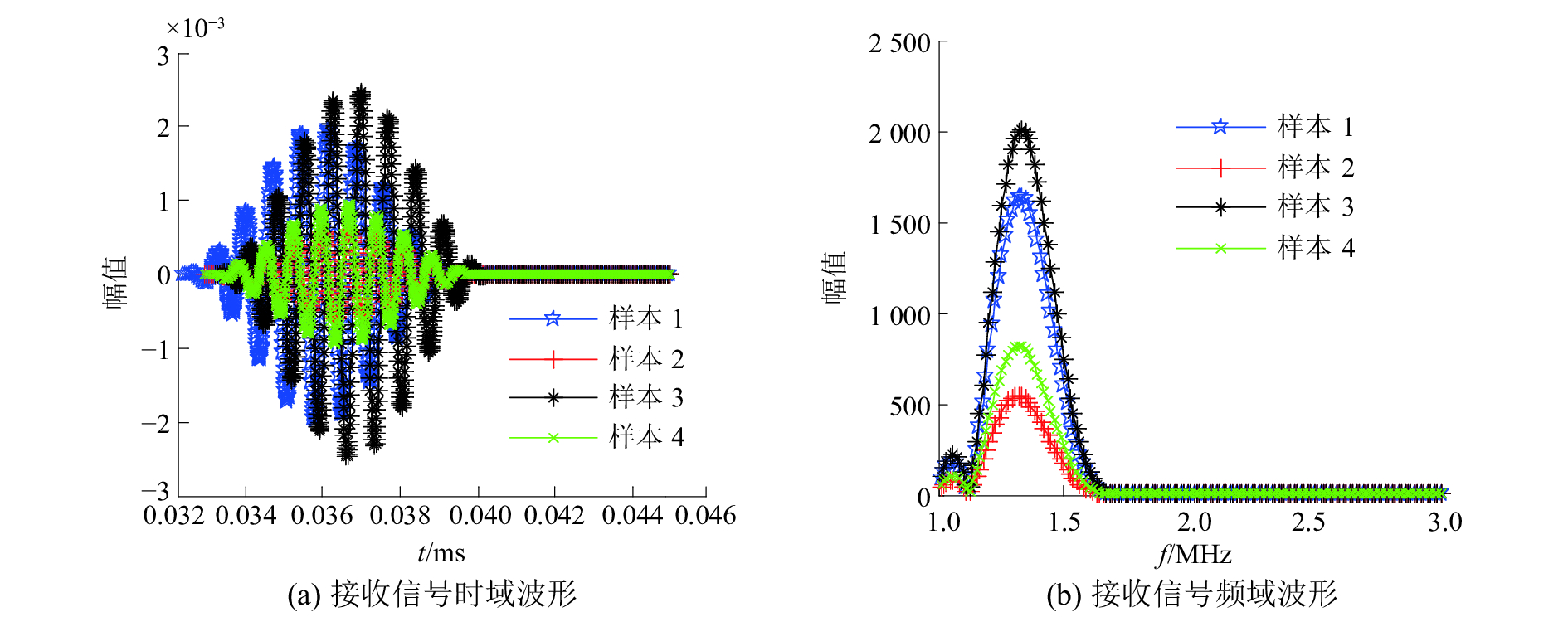Download: 图 7 三层组织的接收信号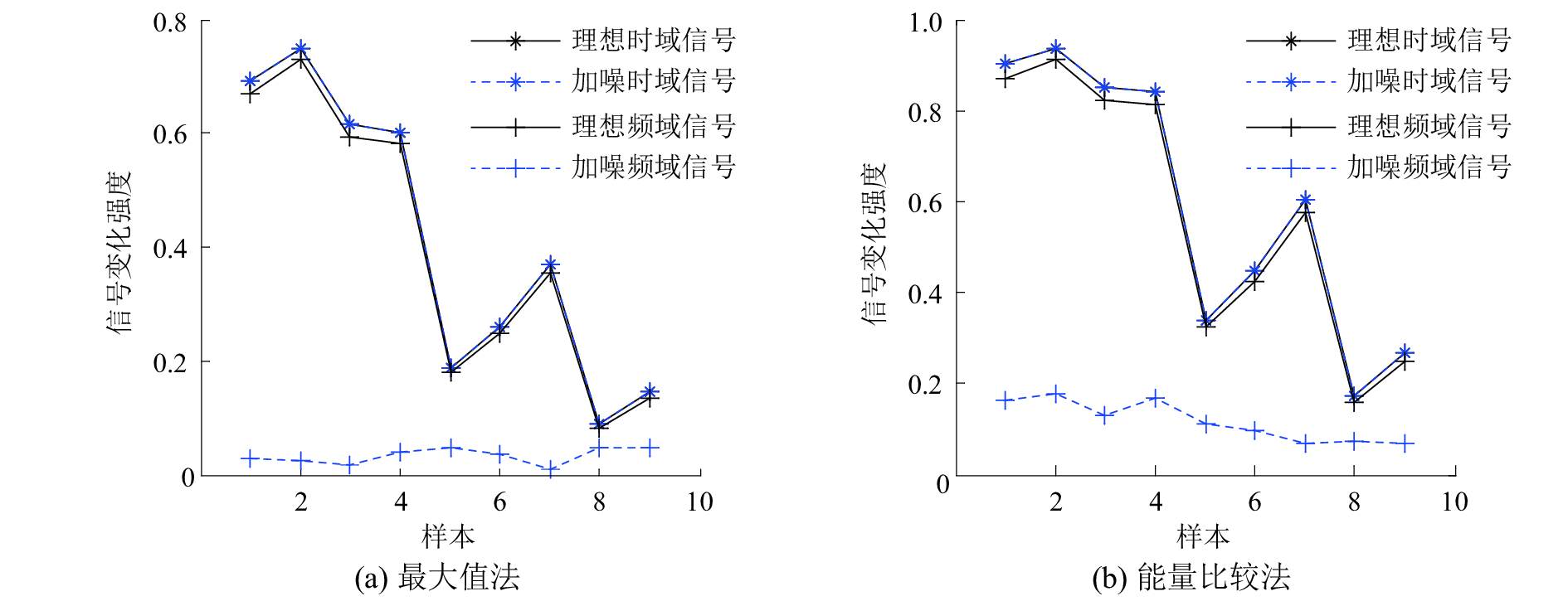Download: 图 8 信号变化示意图（三层）
4 结论

1）本文针对生物组织微结构成分的信号检测问题，采用时域、频域信号检测对比分析，目的是为了比较两者对于组织成分变化的敏感程度。

2）对接收信号进行比较，涉及到信号的提取问题，本文采用简便直观的最大值法和稳定的能量比较法对信号进行提取比较。结果表明，当生物组织成分发生变化时，时域信号的变化程度更强，对组织微结构变化识别度更高，有利于识别组织异变等变化。

3）最大值法与能量法相比较，能量比较法对于信号的测量更稳定，对信号变化感知度更强，有利于反映信号的异常变化。

3）同时，最大值法与能量法相比较，能量比较法对于信号的测量更稳定，对信号变化感知度更强，有利于反映信号的异常变化。

4）根据时域信号在检测精度方面的价值，在生物组织的超声诊断中，采用时域信号对组织病变进行检测，能量比较法对信号进行测量，利用超声在病变信号与正常组织信号的差异，形成能量灰度不同的图像，提高超声诊断的准确性。

  GONCHARSKY A V, ROMANOV S Y, SERYOZHNIKOV S Y. A computer simulation study of soft tissue characterization using low-frequency ultrasonic tomography[J]. Ultrasonics, 2016, 67: 136-150. DOI:10.1016/j.ultras.2016.01.008 (0)  WEAR K A. The effects of frequency-dependent attenuation and dispersion on sound speed measurements: applications in human trabecular bone[J]. IEEE transactions on ultrasonics, ferroelectrics, and frequency control, 2000, 47(1): 265-273. DOI:10.1109/58.818770 (0)  白航, 赵拥军, 胡德秀, 等. 基于改进时频分析方法的雷达信号瞬时频率估计[J]. 信号处理, 2012, 28(2): 257-263. DOI:10.3969/j.issn.1003-0530.2012.02.016 (0)  BERNARD S, KAZEMIRAD S, CLOUTIER G. A frequency-shift method to measure shear-wave attenuation in soft tissues[J]. IEEE transactions on ultrasonics, ferroelectrics, and frequency control, 2017, 64(3): 514-524. DOI:10.1109/TUFFC.2016.2634329 (0)  LABYED Y, BIGELOW T A. A theoretical comparison of attenuation measurement techniques from backscattered ultrasound echoes[J]. The journal of the acoustical society of America, 2011, 129(4): 2316-2324. DOI:10.1121/1.3559677 (0)  ZHENG Liying, SHI Daming. Maximum amplitude method for estimating compact fractional Fourier domain[J]. IEEE signal processing letters, 2010, 17(3): 293-296. DOI:10.1109/LSP.2009.2038511 (0)  CHEN Ting, SHIN J, HUANG Lianjie. Ultrasound transmission attenuation tomography using energy-scaled amplitude ratios[C]//Proceedings of SPIE 9790, Medical Imaging: Ultrasonic Imaging and Tomography. San Diego, California, USA, 2016. (0)  CUCCARO R, MUSACCHIO C, GIULIANO ALBO P A, et al. Acoustical characterization of polysaccharide polymers tissue-mimicking materials[J]. Ultrasonics, 2015, 56: 210-219. DOI:10.1016/j.ultras.2014.03.018 (0)  PÉREZ-LIVA M, HERRAIZ J L, UDIAS J M, et al. Full-wave attenuation reconstruction in the time domain for ultrasound computed tomography[C]//Proceedings of the 13th International Symposium on Biomedical Imaging. Prague, Czech Republic, 2016: 710-713. (0)  MOLERO M, SEGURA I, APARICIO S, et al. On the measurement of frequency-dependent ultrasonic attenuation in strongly heterogeneous materials[J]. Ultrasonics, 2010, 50(8): 824-828. DOI:10.1016/j.ultras.2010.04.006 (0)  赵强. 医学影像设备[M]. 上海: 第二军医大学出版社, 2000. (0)  CULJAT M O, GOLDENBERG D, TEWARI P, et al. A review of tissue substitutes for ultrasound imaging[J]. Ultrasound in medicine & biology, 2010, 36(6): 861-873. (0)